# cyancrow140

Lv8

## University of Mumbai

0 Followers
0 Following
1 Helped
I love to solve the math problem.I am here to solve maths problem and help other as well as to evolve my ability to solve maths sums.

Published210

### Subjects

Algebra63Calculus24Mathematics123
Answer: the given sequence is Geometric sequence and the common difference is ...
Find the greatest common factor for the list of terms.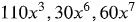The greatest common factor is ⬜

Answer: greatest common divisor of 110x^3, 30x^6 and 60x^7 is 10x^3.Step-by-st...
Find the greatest common factor.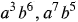The greatest common factor (GCF) of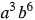and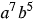is ⬜

Answer: the greatest common divisor of a^3b^6 and a^7b^5 is a^3b^5Step-by-step...
Complete the factoring.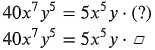Answer: The required factor is 8(x^2)(y^4)Step-by-step explanation:Since, 40x^...
Answer: The rate of interest is 5%Step-by-step explanation:Here, Amount invest...
Answer: 3/4 divided by 2=3/8Step-by-step explanation:3/4 divided by 2 =(3/4)/2...
Answer: (-6-4) divided by (-5)=2Step-by-step explanation:(-6-4) divide by (-5)...
Answer: 7 divided by 13=7/13Step-by-step explanation:7 divided by 13 =7/13 As ...
Answer: 2/3 divided by half =4/3Step-by-step explanation:2/3 divided by half =...
Answer: Thus, 24 divided by 3=8Step-by-step explanation:Since, 24 divided by 3...
Answer: 3/4 divided by 2=3/8Step-by-step explanation:3/4 divided by 2 =(3/4)/2...
Answer: 3/4 divided by 2=3/8Step-by-step explanation:3/4 divided by 2 =(3/4)/2...
Answer: Thus, 12 divided by 1/3=36Step-by-step explanation:Since, 12 divided b...
Answer: Thus the required answer is, 42 divided by 3/4 =56Step-by-step explana...
Answer: 4 1/3 divide 1 1/6=26/7Step-by-step explanation:4 1/3 divide 1 1/6 = (...
Answer: Thus, (2,6,7)-(1,7,3)=(1,-1,4)Step-by-step explanation:Since, (2, 6, 7...
Answer: Thus, 2/3 divide (5/7 divide 3/6)+1/2=29/30Step-by-step explanation:Si...
Answer: The required answer is, (7, 2, -6)-(7, 2, -4)=(0, 0, -2)Step-by-step e...
Answer: The required answer is (2, 2, 7)-(-5, 5, -4)=(7, -3, 11)Step-by-step e...
Answer: 8+1÷6×3=17/2Step-by-step explanation:Since, the given equation is 8+1÷...

Find the term that must be added to the equation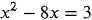to make it into a perfect square.

◯ A. -3

B. 32

C. 16

◯ D. 64

Answer: 16 is the number must be added to the equation to make it perfect Step...
Answer: XV=15Step-by-step explanation:Since, X=10 and V=5 Thus, XV=10+5 =15
Answer: 32 tens=320Step-by-step explanation:Since, 32 tens can be written as 3...
Answer: 28 tens=280Step-by-step explanation:Since, 28 tens can be written as 2...
Answer: 30 tens=300Step-by-step explanation:Since. 30 tens can be written as 3...
Answer: 20 tens=200Step-by-step explanation:Since, 20 tens can be written as 2...
Answer: 50 tens=500Step-by-step explanation:Since, 50 tens can be written as 5...